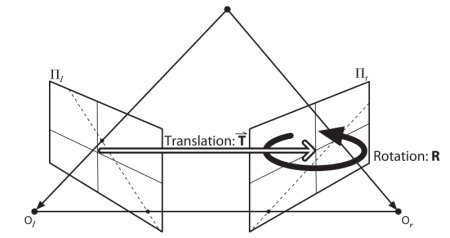# 对极几何¶

### 基础概念¶

$O$$O'$是相机中心。从上面给出的设置中，您可以看到在点处的左侧图像上可以看到右侧摄像机$O'$的投影。它称为**极点**。极点是穿过相机中心和图像平面的线的交点。左摄像机的极点也同理。在某些情况下，您将无法在图像中找到极点，它们可能位于图像外部（这意味着一个摄像机看不到另一个摄像机）。### 代码¶

import numpy as np
import cv2 as cv
from matplotlib import pyplot as plt
img1 = cv.imread('myleft.jpg',0)  #索引图像 # left image
img2 = cv.imread('myright.jpg',0) #训练图像 # right image
sift = cv.SIFT()
# 使用SIFT查找关键点和描述符
kp1, des1 = sift.detectAndCompute(img1,None)
kp2, des2 = sift.detectAndCompute(img2,None)
# FLANN 参数
FLANN_INDEX_KDTREE = 1
index_params = dict(algorithm = FLANN_INDEX_KDTREE, trees = 5)
search_params = dict(checks=50)
flann = cv.FlannBasedMatcher(index_params,search_params)
matches = flann.knnMatch(des1,des2,k=2)
good = []
pts1 = []
pts2 = []
# 根据Lowe的论文进行比率测试
for i,(m,n) in enumerate(matches):
if m.distance < 0.8*n.distance:
good.append(m)
pts2.append(kp2[m.trainIdx].pt)
pts1.append(kp1[m.queryIdx].pt)


pts1 = np.int32(pts1)
pts2 = np.int32(pts2)
F, mask = cv.findFundamentalMat(pts1,pts2,cv.FM_LMEDS)
# 我们只选择内点


def drawlines(img1,img2,lines,pts1,pts2):
''' img1 - 我们在img2相应位置绘制极点生成的图像
lines - 对应的极点 '''
r,c = img1.shape
img1 = cv.cvtColor(img1,cv.COLOR_GRAY2BGR)
img2 = cv.cvtColor(img2,cv.COLOR_GRAY2BGR)
for r,pt1,pt2 in zip(lines,pts1,pts2):
color = tuple(np.random.randint(0,255,3).tolist())
x0,y0 = map(int, [0, -r/r ])
x1,y1 = map(int, [c, -(r+r*c)/r ])
img1 = cv.line(img1, (x0,y0), (x1,y1), color,1)
img1 = cv.circle(img1,tuple(pt1),5,color,-1)
img2 = cv.circle(img2,tuple(pt2),5,color,-1)
return img1,img2


# 在右图（第二张图）中找到与点相对应的极点，然后在左图绘制极线
lines1 = cv.computeCorrespondEpilines(pts2.reshape(-1,1,2), 2,F)
lines1 = lines1.reshape(-1,3)
img5,img6 = drawlines(img1,img2,lines1,pts1,pts2)
# 在左图（第一张图）中找到与点相对应的Epilines，然后在正确的图像上绘制极线
lines2 = cv.computeCorrespondEpilines(pts1.reshape(-1,1,2), 1,F)
lines2 = lines2.reshape(-1,3)
img3,img4 = drawlines(img2,img1,lines2,pts2,pts1)
plt.subplot(121),plt.imshow(img5)
plt.subplot(122),plt.imshow(img3)
plt.show()


### 练习¶

1. 一个重要的主题是相机的前移。然后，将在两个位置的相同位置看到极点，并且从固定点出现极点。 请参阅此讨论。
2. 基本矩阵估计对匹配，离群值等的质量敏感。如果所有选定的匹配都位于同一平面上，则情况会变得更糟。检查此讨论。